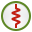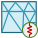# LE Card

This card specifies complex series and parallel circuits applied to an edge between surface triangles.

On the Source/Load tab, in the Loads / networks group, click theLoad icon. From the drop-down list, click theLoad edge (LE) icon.

## Parameters:

Define/replace a load at an edge
Define a load with the following parameters.
Remove all LE type loads previously defined
This LE card does not define a load, but rather all previously defined LE loads are deleted. All the other input parameters of this card are ignored.
Load edge between regions with multiple labels
The edge between different regions is loaded with a complex impedance. The following parameters apply when this item is checked:
Maximum number of labels on a side
his indicates the maximum number of different labels used on either side of the load. Increasing this value will add rows to the table for the labels.
Negative side
The Labels of triangles on the one side of the load.
Positive side
The Labels of triangles on the other side of the load.
Load an edge connected to ground/UTD
Load the triangles with specified labels that are connected to a UTD surface or to a PEC ground plane (as specified with a BO or GF card). The following parameters apply when this item is checked:
Maximum number of labels on a side
This indicates the maximum number of different labels used on either side of the load. Increasing this value will add rows to the table for the labels.
Meshed surface represents positive feed side
If selected, the Positive side of the Labels of triangles is connected to ground.
If not selected, the Negative side of the Labels of triangles is connected to ground.
Load microstrip edge between two points
This is a special microstrip port load. The load is placed on all edges on the line between two points (previously specified with DP cards) entered into the dialog. A GF card with a conducting ground plane must be present. The following parameters apply when this item is checked:
Start point of edge
The start point (not label) of the edge.
End point of edge
The end point (not label) of the edge.
Meshed surface represents positive feed side
If selected, the positive side of the microstrip edge between two points is connected to ground.
If not selected, the negative side of the microstrip edge between the points is connected to ground.
Complex impedance
The real and imaginary part of the complex impedance in $\Omega$ .
Series circuit
The resistor value in $\Omega$ , inductor value in Henry and the capacitor value in Farad to be added as a series circuit.
Parallel circuit
The resistor value in $\Omega$ , inductor value in Henry and the capacitor value in Farad to be added as a parallel circuit.
SPICE circuit
Specify the name of a one-port SPICE circuit to define a load between two pins. Define the SPICE circuit using the SC card.
Touchstone file
Specify a one-port Touchstone file (.s1p, .z1p, .y1p) to define a load.
Note: If the load is added to a port that has a voltage source, the load is placed in series with the voltage source.

See also the AE card for the excitation of such an edge. As shown in the figure above, the edge can consist of several single edges between regions specified by labels. (See the AE card for a discussion on the allowed configurations.) Alternatively the edge can be along a connection between triangles and a polygonal plate or a PEC ground plane, or it can be a microstrip feed line port. The impedance Z applies to the complete edge (all the single edges in parallel). The LE card can be combined with the AE card to specify both an impedance and a voltage source over the edge.

Note that the edge between the triangles does not need to be straight. One may, for example, specify a resistive connection between two half cylinders.